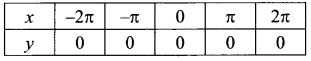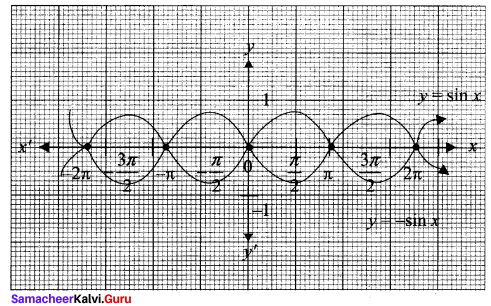## Tamilnadu Samacheer Kalvi 11th Maths Solutions Chapter 1 Sets Ex 1.4

11th Maths Exercise 1.4 Solutions Question 1.
For the curve y = x3 given in Figure, draw
(i) r = -x3
(ii) y = x3 + 1
(iii) y = x3 – 1
(iv) y = (x + 1)3 with the same scale.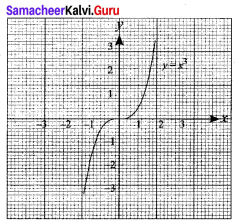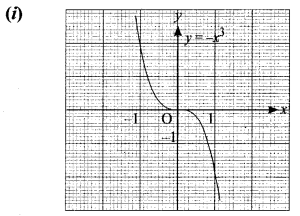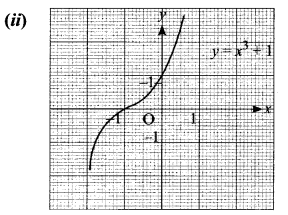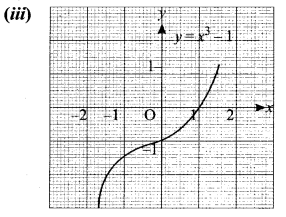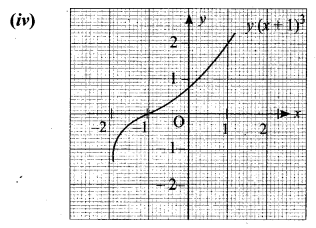Solution:
(i) It is the reflection on y axis
(ii) The graph of y = x3 + 1 is shifted upward to 1 unit.
(iii) The graph of y = x3 – 1 is shifted downward to 1 unit.
(iv) The graph of y = (x + 1)3 is shifted to the left for 1 unit.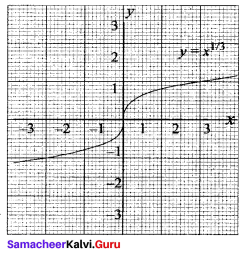11th Maths Exercise 1.4 Answers Question 2.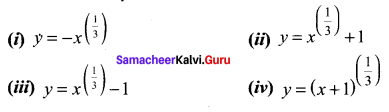Solution: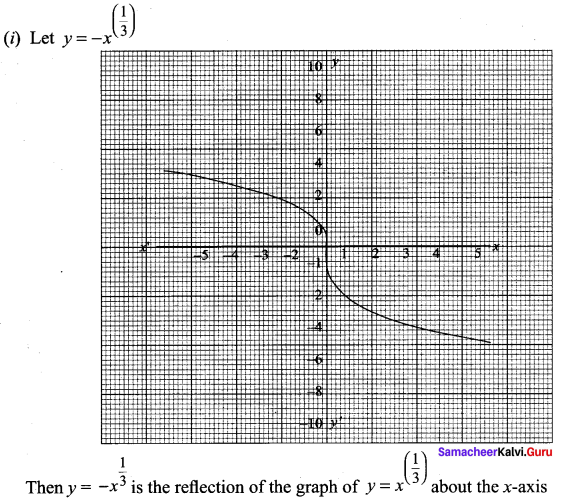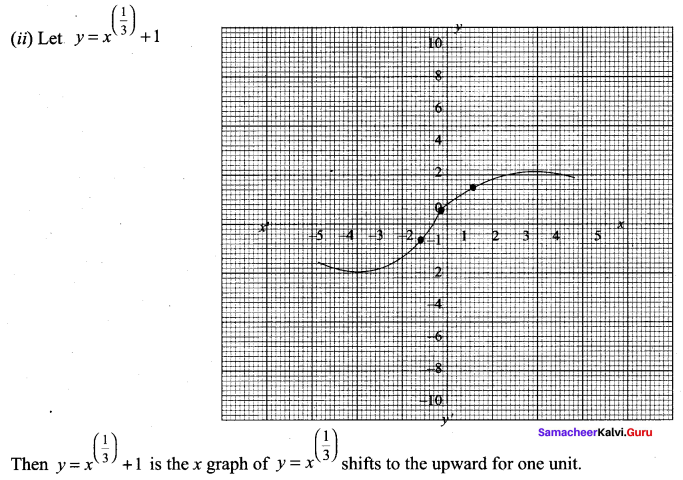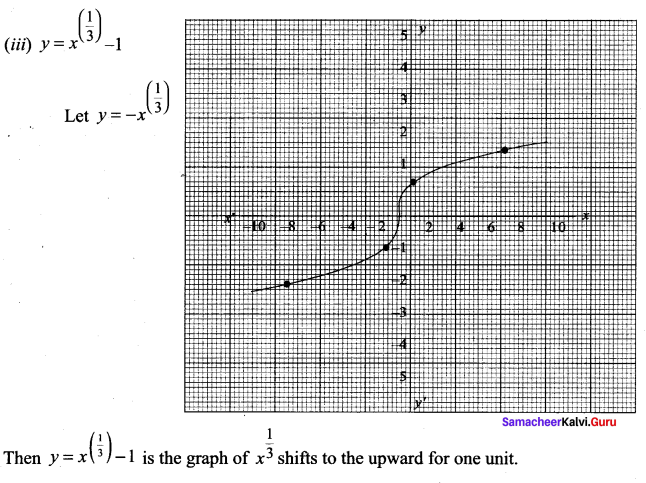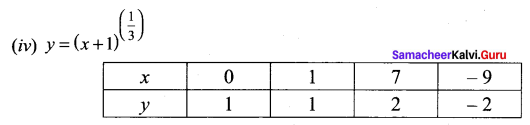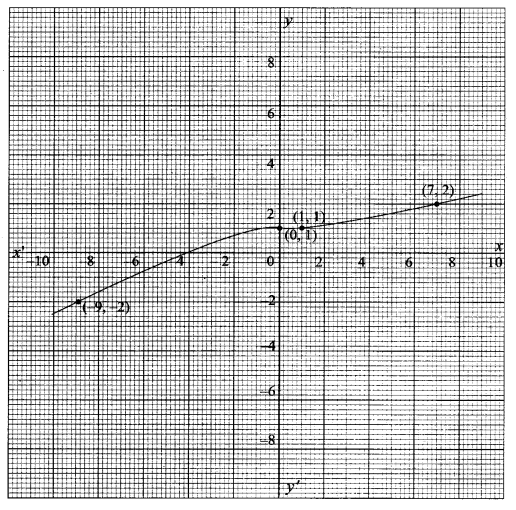11th Maths Exercise 1.4 Question 3.
Graph the functions f(x) = x3 and g(x) = $$\sqrt{x}$$ on the same coordinate plane. Find fog and graph it on the plane as well. Explain your results.
Solution: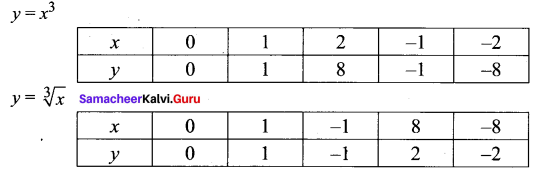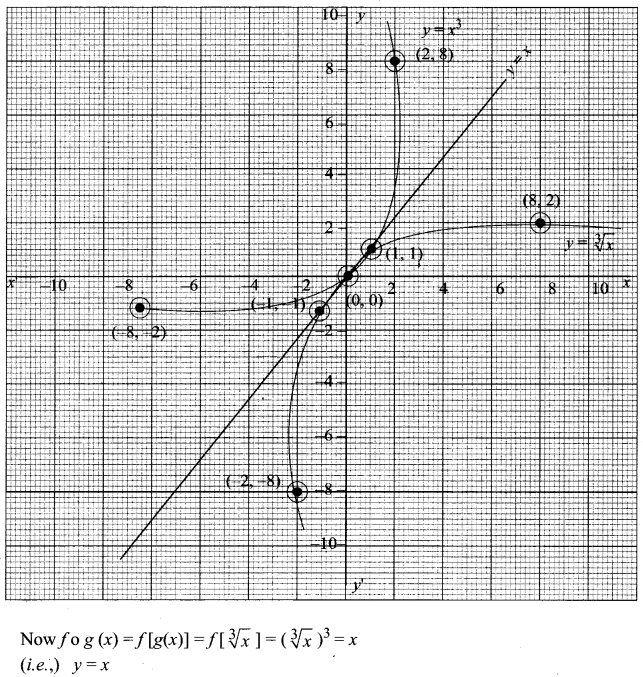Exercise 1.4 Class 11 Maths Solutions Question 4.
Write the steps to obtain the graph of the function y = 3(x – 1)2 + 5 from the graph y = x2.
Solution:
Draw the graph of y = x2
To get y = (x – 1)2 we have to shift the curve 1 unit to the right.
Then we have to draw the curve y = 3(x – 1)2 and finally we have to draw y = 3(x – 1)2 + 5

Samacheer Kalvi Guru 11th Maths Question 5.
From the curve y = sin x, graph the functions
(i) y = sin(-x)
(ii) y = -sin(-x)
(iii) y = sin ($$\frac{\pi}{2}$$ + x) which is cos x
(iv) y = sin ($$\frac{\pi}{2}$$ – x) which is also cos x (refer trigonometry)
Solution:
First we have to draw the curve y = sin x

(i) y = sin (-x) = – sin x = f(x)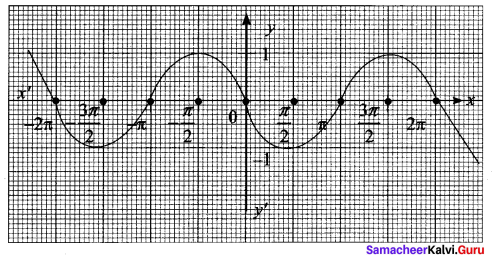(ii) y = -sin(-x) = -[-sin x] = sin x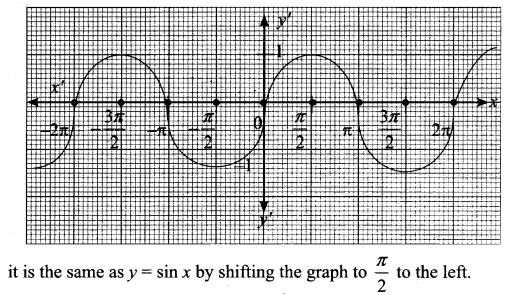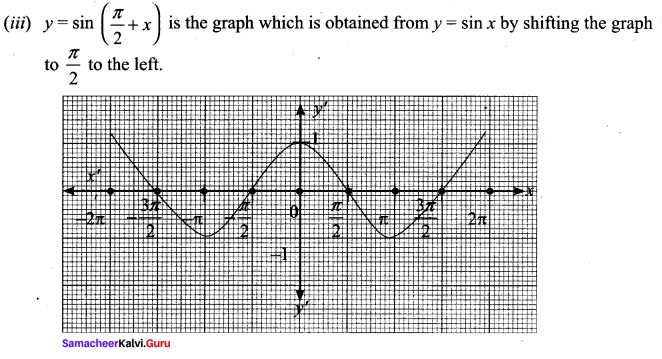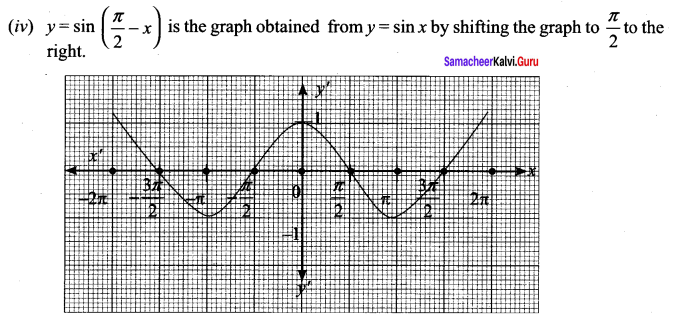Maths Solution Class 11 Samacheer Kalvi Question 6.
From the curve y = x, draw
(i) y = -x
(ii) y = 2x
(iii) y = x + 1
(iv) y = $$\frac{1}{2}$$x + 1
(v) 2x + y + 3 = 0. 2
Solution:
y = x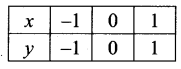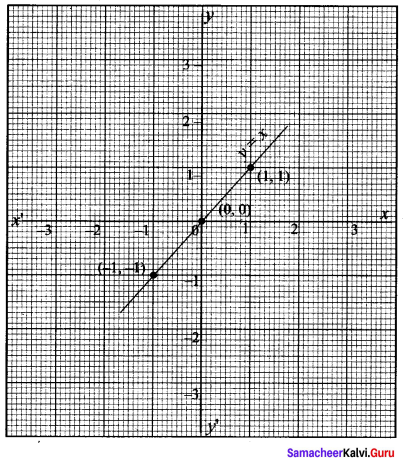(i) y = -x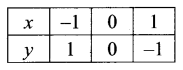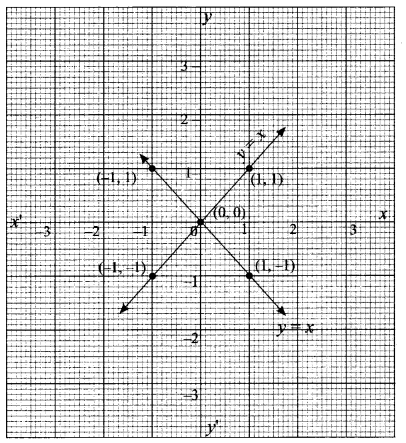(ii) y = 2x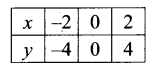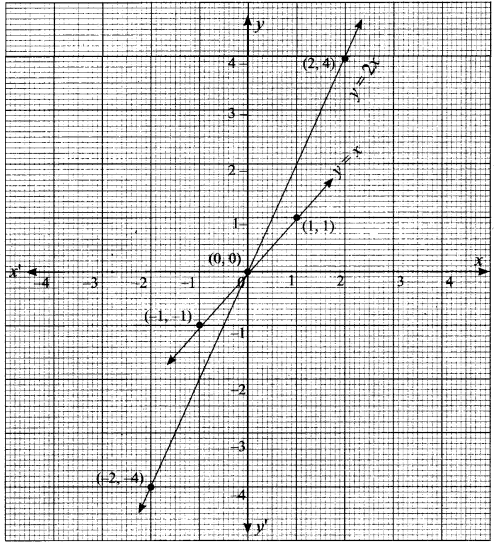y = 2x the graph moves away the x-axis, as multiplying factor is 2 which is greater than one.

(iii) y = 2x + 1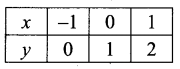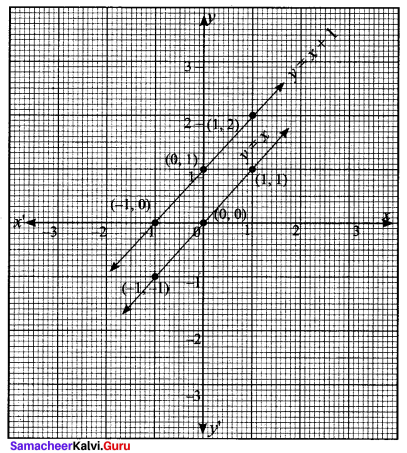(iv) y = 1/2x + 1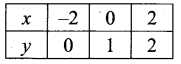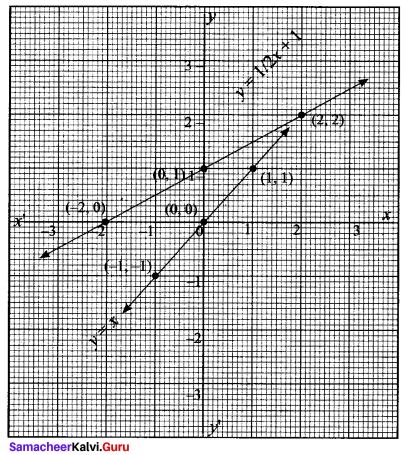y = $$\frac{1}{2}$$x moves towards x – axis by a side factor $$\frac{1}{2}$$ which is less than y = $$\frac{1}{2}$$x + 1 upwards by 1 unit.

(v) y = -2x – 3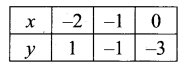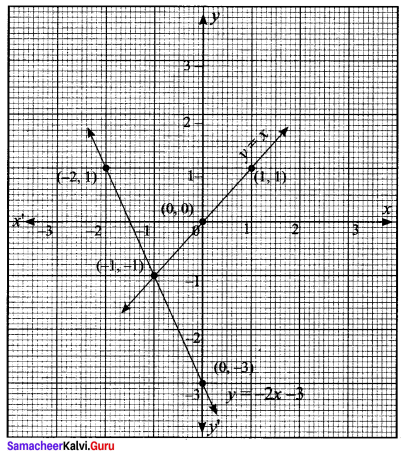Samacheer Kalvi.Guru 11th Maths Question 7.
From the curve y = |x|, draw
(i) y= |x – 1| + 1
(ii) y = |x + 1| – 1
(iii) y = |x + 2| – 3.
Solution:
Given, y = |x|
If y = x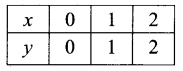If y = -x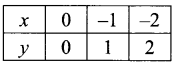(i) y = |x – 1| + 1
y = x – 1 + 1
y = -x + 1 + 1 = x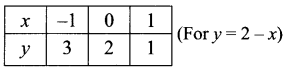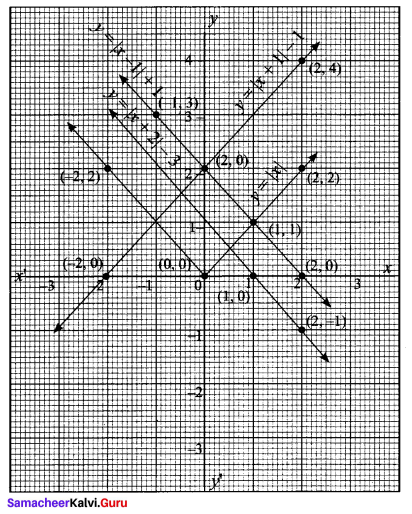(ii) y = |x + 1| – 1
y = x + 1 – 1 = x
y = -x -1 – 1
y = -x – 2
y = – (x + 2)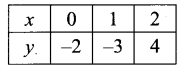(iii) y = |x + 2| – 3
y = x + 2 – 3 ⇒ x – 1
y = -x – 2 + 3 = 1 – x
y = -(x – 1)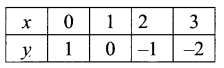Question 8.
From the curves = sin x, draw y = sin |x| (Hint: sin(-x) = -sin x.)
Solution:
y = sin |x|
∴ y = sin x
∴ y = sin (-x) = – sin x
y = – sin x